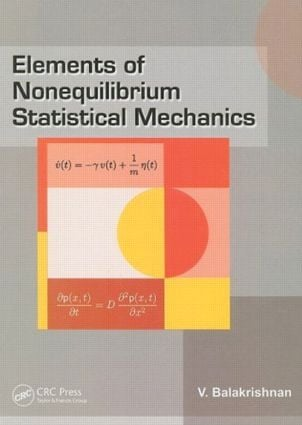Elements of Nonequilibrium Statistical Mechanics

1st Edition

CRC Press

314 pages

Hardback: 9781420074192
pub: 2008-03-04
\$140.00
x

FREE Standard Shipping!

Description

This book deals with the basic principles, techniques, and advances being made--both experimentally and theoretically--in the study of nonequilibrium phenomena, particularly in statistical physics, chemical physics, biological physics, complex systems, and several other areas. Accessible to senior undergraduate students and post-graduate students, this self-contained book offers a choice of topics that enables them to form a coherent picture of the subject. Coverage includes equations and formulas for calculating distribution, diffusion, and dynamic mobility under different conditions. The book concludes with a generalized Langevin equation and the power spectrum of pulse sequences.

Introduction

The Langevin Equation

The Fluctuation-dissipation relationship

The Velocity autocorrelation function

Markov Processes

The Fokker-Planck equation and the Ornstein-Uhlenbeck distribution

Mean-squared displacement from the Langevin equation

The Fokker Planck equation in phase space

The diffusion equation

Brownian motion

Diffusion in a force field

The Kubo-Green formula

The dynamic mobility as a generalized susceptibility

Power spectrum of a random process

The generalized Langevin equation

Power spectrum of a random pulse sequence

Bibliography

Index